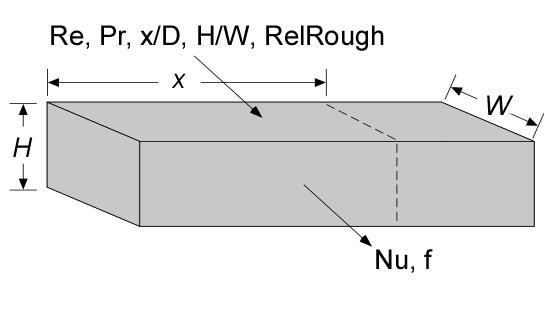Contents - Index

DuctFlow_ND_localProcedure DuctFlow_ND_local(Re, Pr, xoverD_h, Aspect, relRough: Nusselt_T_x,Nusselt_H_x, f_x) returns the local Nusselt number assuming constant temperature wall and constant heat flux for a given flow condition in a rectangular duct characterized by Reynolds and Prandtl numbers, local position/hydraulic diameter, aspect ratio, and relative roughness.  The procedure also returns the friction factor for these conditions.   The procedure can be used for simultaneous thermal and hydrodynamic developing flow or fully-developed flow in the laminar, transitional or turbulent flow regimes.

Inputs:

Re - Reynolds number based on properties evaluated at the bulk average temperature

Pr - Prandtl number of the fluid evaluated at the bulk average temperature

xoverD_h - ratio of axial position to hydraulic diameter.

Aspect - ratio of the minimum to maximum dimension of duct.  This value should be <=1

RelRough - the ratio of the dispersions on the wall of the duct to the duct hydraulic diameter (must be between 0 and 0.05)

Outputs:

Nusselt_T_x -local Nusselt number assuming a constant wall temperature

Nusselt_H_x - local Nusselt number assuming a constant wall heat flux

f_x -  apparent local friction factor [-]

Notes:

This procedure calls DuctFlow_N to obtain the average Nusselt numbers and friction factor.  See that routine or Section 5.2.4 of Nellis and Klein for additional information.

Two Nusselt numbers are returned.  Nusselt_T_x corresponds to a constant temperature wall and Nusselt_H_x corresponds to a constant heat flux.  These two values will be the same for turbulent flow.  For laminar flow, they provide upper and lower bounds on the Nusselt number.

The procedure assumes simultaneous development of the thermal and hydrodynamic boundary layers; however, the results for a thermally developing/hydrodynamically developed condition can be obtained by calling the procedure with a large Prandtl number.

If  xoverD is less than 0.1, it is set to 0.1..

Example:

Re=4500

Pr=2

Aspect=0.25

xoverD_h=20

relRough=0

call DuctFlow_ND_local(Re, Pr, xoverD_h, Aspect, RelRough: Nusselt_T_x, Nusselt_H_x, f_x)

{Solution:

f_x=0.03999

Nusselt_H_x=23.05 [-]

Nusselt_T_x=23.05 [-]}

Internal Flow Index